Courses

# Doc: Dielectrics and Polarization Class 12 Notes | EduRev

## Class 12 : Doc: Dielectrics and Polarization Class 12 Notes | EduRev

The document Doc: Dielectrics and Polarization Class 12 Notes | EduRev is a part of the Class 12 Course Physics Class 12.
All you need of Class 12 at this link: Class 12

9. FORCE BETWEEN THE PLATES OF A CAPACITOR

In a capacitor as plates carry equal and opposite charges, there is a force of attraction between the plates. To calculate this force, we use the fact that the electric field is conservative and in a conservative field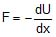. In case of parallel plate capacitor: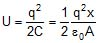[as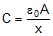]

So,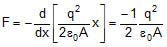The negative sign implies that the force is attractive.

10. Capacitors with dielectric :

(i) In absence of dielectric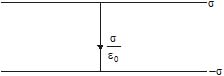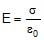(ii) When a dielectric fills the space between the plates then molecules having dipole moment align themselves in the direction of electric field.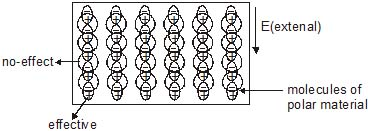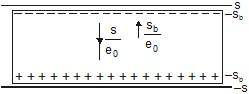sb = induced charge density (called bound charge because it is not due to free electrons).

* For polar molecules dipole moment ¹ 0

* For non-polar molecules dipole moment = 0

(iii) Capacitance in the presence of dielectric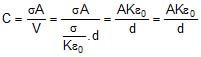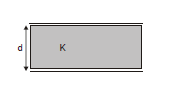Here capacitance is increased by a factor K.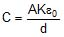(iv) Polarisation of material :

When a non-polar substance is placed in an electric field then dipole moment is induced in the molecule. This induction of dipole moment is called polarisation of material. The induced charge also produces electric field.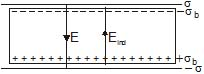σb = induced (bound) charge density.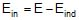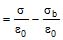It is seen the ratio of electric field between the plates in absence of dielectric and in presence of dielectric is constant for a material of dielectric. This ratio is called 'Dielectric constant' of that material. It is represented by er or k.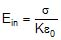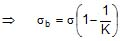(v) If the medium is not filled between the plates completely then electric filed will be as shown in figure.

Case : (1)

The total electric field produced by bound induced charge on the dielectric outside the slab is zero because they cancel each other.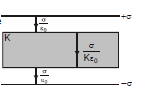Case : (2)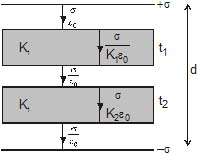so potential difference between plates

v =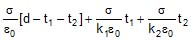so equivalent capacitance

C =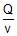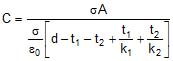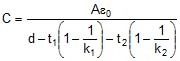10.1 Introduction of a Dielectric slab of dielectric constant K between the plates

(a) When the battery is disconnected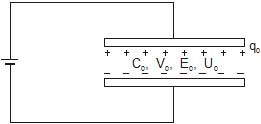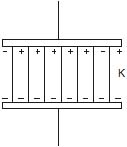Let q0, C0, V0, E0 and U0 represents the charge, capacity, potential difference, electric field and energy associated with charged air capacitor respectively. With the introduction of a dielectric slab of dielectric constant K between the plates and the battery disconnected.

(i) Charge remains constant, i.e., q = q0, as in an isolated system charge is conserved.

(ii) Capacity increases, i.e., C = KC0, as by the presence of a dielectric capacity becomes K times.

(iii) Potential difference between the plates decreases, i.e.,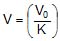, as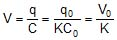[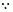q = q0 and C = KC0]

(iv) Field between the plates decreases, i.e.,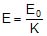, as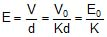[as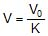]

and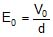(v) Energy stored in the capacitor decreases i.e.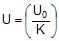, as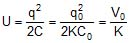(as q = q0 and C = KC0]

(b) When the battery remains connected (potential is held constant)

(i) Potential difference remains constant, i.e., V = V0, as battery is a source of constant potential difference.

(ii) Capacity increases, i.e., C = KC0, as by presence of a dielectric capacity becomes K times.

(iii) Charge on capacitor increases, i.e., q = Kq0, as

q = CV = (KC0)V = Kq0 [q0 = C0V]

(iv) Electric field remains unchanged, i.e., E = E0, as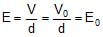[as V = V0 and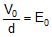]

(v) Energy stored in the capacitor increases, i.e., U = KU0, as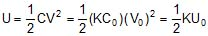[as C = KC0 and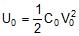]

Ex.41 A parallel plate air capacitor is made using two square plates each of side 0.2 m, spaced 1 cm apart. It is connected to a 50V battery.

(a) What is the capacitance ?

(b) What is the charge on each plate ?

(c) What is the energy stored in the capacitor ?

(d) What is the electric field between the plates ?

(e) If the battery is disconnected and then the plates are pulled apart to a separation of 2 cm, what are the answers to the above parts ?

Sol. (a)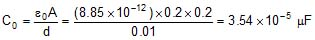(b) Q0 = C0V0 = 3.54 × 10-5 × 50 = 1.77 × 10-3 mC

(c)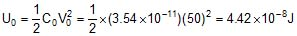(d)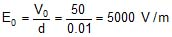(e) If the battery is disconnected the charge on the capacitor plates remains constant while the potential difference between the plates can change.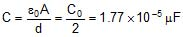Q = Q0 = 1.77 × 10-3 mC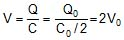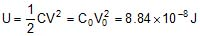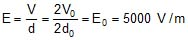Ex.42 In the last illustration, suppose that the battery is kept connected while the plates are pulled apart. What are the answers to the parts (a), (b), (c) and (d) in that case ?

Sol. If the battery is kept connected, the potential difference across the capacitor plates always remains equal to the emf of battery and hence is constant.

V = V0 = 50V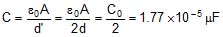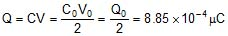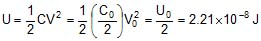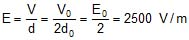Ex.43 A parallel plate capacitor has plates of area 4 m2 separated by distance of 0.5 mm. The capacitor is connected across a cell of emf 100 V.

(a) Find the capacitance, charge and energy stored in the capacitor.

(b) A dielectric slab of thickness 0.5 mm is inserted inside this capacitor after it has been disconnected from the cell. Find the answers to part (a) if K = 3.

Sol. (a)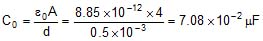Q0 = C0V0 = (7.08 × 10-2 × 100)mC = 7.08 mC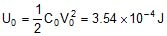(b) As the cell has been disconnected

Q = Q0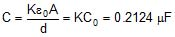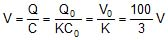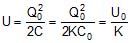= 118 × 10-6 J

Ex.44 If a dielectric slab of thickness t and area A is inserted in between the plates of a parallel plate capacitor of plate area A and distance between the plates d (d > t) then find out capacitance of system. What do you predict about the dependence of capacitance on location of slab ?

Sol.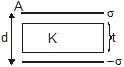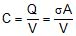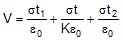(t1  t2 = d - t)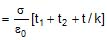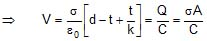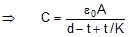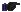* Capacitance does not depend upon the position of dielectric (it can be shifted up or down & still capacitance does not change).

* If the slab is of metal then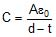Ex.45 Find out capacitance between A and B if two dielectric slabs of dielectric constant K1 and K2 of thickness d1 and d2 and each of area A are inserted between the plates of parallel plate capacitor of plate area A as shown in figure.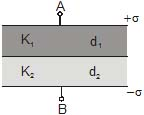Sol.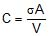;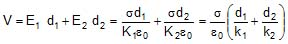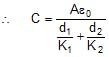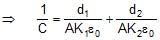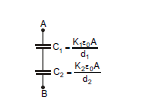This formula suggests that the system between A and B can be considered as series combination of two capacitors.

Ex.46 Find out capacitance between A and B if two dielectric slabs of dielectric constant K1 and K2 of area A1 and A2 and each of thickness d are inserted between the plates of parallel plate capacitor of plate area A as shown in figure.

Sol.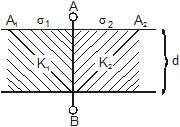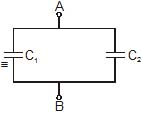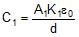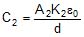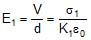;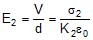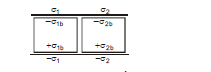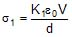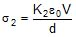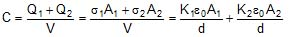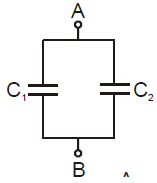The combination is equivalent to :

Therefore, C = C1  C2

Ex.47 Find out capacitance between A and B if three dielectric slabs of dielectric constant K1 of area A1 and thickness d1 K2 of area A2 and thickness d2 and K3 of area A2 and thickness d3 are inserted between the plates of parallel plate capacitor of plate area A as shown in figure. (Given distance between the two plates d = d1  d2)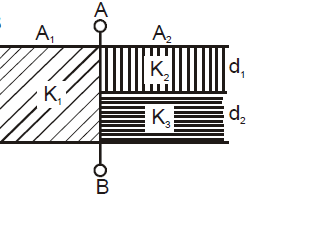Sol. It is equivalent to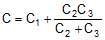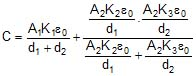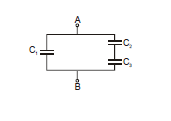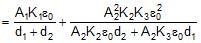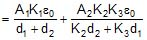Ex.48 A dielectric of constant K is slipπεd between the plates of parallel plate condenser in half of the space as shown in the figure. If the capacity of air condenser is C, then new capacitance between A and B will be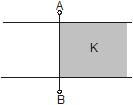(A)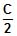(B)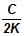(C)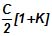(D)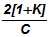Sol. This system is equivalent to two capacitors in parallel with area of each plate

Offer running on EduRev: Apply code STAYHOME200 to get INR 200 off on our premium plan EduRev Infinity!

,

,

,

,

,

,

,

,

,

,

,

,

,

,

,

,

,

,

,

,

,

;